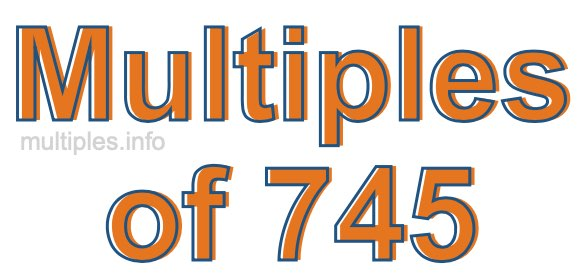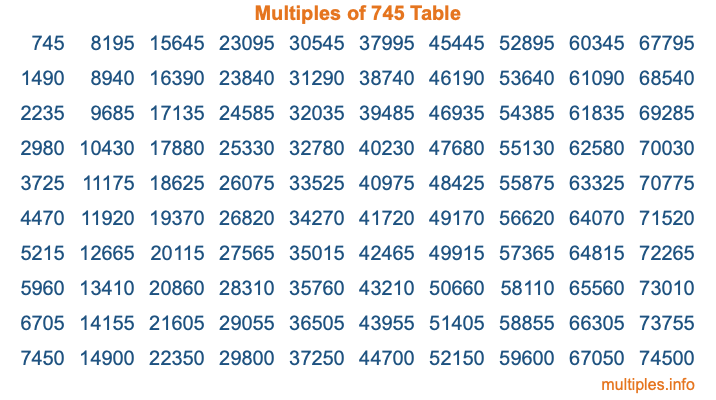Multiples of 745Welcome to the Multiples of 745 page. Here we will first teach you everything you will ever need to know about the multiples of 745, and then give you a study guide summary of everything we taught you to make sure you remember it all. Use this page to look up facts and learn information about the multiples of 745. This page will make you a multiples of seven hundred forty-five expert!

Definition of Multiples of 745
Multiples of 745 are all the numbers that when divided by 745 equal an integer. Each of the multiples of 745 are called a multiple. A multiple of 745 is created by multiplying 745 by an integer.

Therefore, to create a list of multiples of 745, you start with 1 multiplied by 745, then 2 multiplied by 745, then 3 multiplied by 745, and so on for as long as you want. Thus, the list of the first five multiples of 745 is 745, 1490, 2235, 2980, and 3725. To see a larger list of multiples of 745, see the printable image of Multiples of 745 further down on this page. We also have a category where you can choose any nth multiple of 745.

Multiples of 745 Checker
The Multiples of 745 Checker below checks to see if any number of your choice is a multiple of 745. In other words, it checks to see if there is any number (integer) that when multiplied by 745 will equal your number. To do that, we divide your number by 745. If the the quotient is an integer, then your number is a multiple of 745.

Is  a multiple of 745?

Least Common Multiple of 745 and ...
A Least Common Multiple (LCM) is the lowest multiple that two or more numbers have in common. This is also called the smallest common multiple or lowest common multiple and is useful to know when you are adding our subtracting fractions. Enter one or more numbers below (745 is already entered) to find the LCM.

Check out our LCM Calculator if you need more details about the Least Common Multiple or if you need the LCM for different numbers for adding and subtraction fractions.

nth Multiple of 745
As we stated above, 745 is the first multiple of 745, 1490 is the second multiple of 745, 2235 is the third multiple of 745, and so on. Enter a number below to find the nth multiple of 745.

th multiple of 745

Multiples of 745 vs Factors of 745
745 is a multiple of 745 and a factor of 745, but that is where the similarities end. All postive multiples of 745 are 745 or greater than 745. All positive factors of 745 are 745 or less than 745.

Below is the beginning list of multiples of 745 and the factors of 745 so you can compare:

Multiples of 745: 745, 1490, 2235, 2980, 3725, etc.

Factors of 745: 1, 5, 149, 745

As you can see, the multiples of 745 are all the numbers that you can divide by 745 to get a whole number. The factors of 745, on the other hand, are all the whole numbers that you can multiply by another whole number to get 745.

It's also interesting to note that if a number (x) is a factor of 745, then 745 will also be a multiple of that number (x).

Multiples of 745 vs Divisors of 745
The divisors of 745 are all the integers that 745 can be divided by evenly. Below is a list of the divisors of 745.

Divisors of 745: 1, 5, 149, 745

The interesting thing to note here is that if you take any multiple of 745 and divide it by a divisor of 745, you will see that the quotient is an integer.

Multiples of 745 Table
Below is an image of the first 100 multiples of 745 in a table. The table is in chronological order, column by column. The first column has the first ten multiples of 745, the second column has the next ten multiples of 745, and so on.The Multiples of 745 Table is also referred to as the 745 Times Table or Times Table of 745. You are welcome to print out our table for your studies.

Negative Multiples of 745
Although not often discussed or needed in math, it is worth mentioning that you can make a list of negative multiples of 745 by multiplying 745 by -1, then by -2, then by -3, and so on, to get the following list of negative multiples of 745:

-745, -1490, -2235, -2980, -3725, etc.

Multiples of 745 Summary
Below is a summary of important Multiples of 745 facts that we have discussed on this page. To retain the knowledge on this page, we recommend that you read through the summary and explain to yourself or a study partner why they hold true.

There are an infinite number of multiples of 745.

A multiple of 745 divided by 745 will equal a whole number.

745 divided by a factor of 745 equals a divisor of 745.

The nth multiple of 745 is n times 745.

The largest factor of 745 is equal to the first positive multiple of 745.

745 is a multiple of every factor of 745.

745 is a multiple of 745.

A multiple of 745 divided by a divisor of 745 equals an integer.

745 divided by a divisor of 745 equals a factor of 745.

Any integer times 745 will equal a multiple of 745.

Multiples of a Number
Here you can get the multiples of another number, all with the same attention to detail as we did for multiples of 745 on this page.

Multiples of
Multiples of 746
Did you find our page about multiples of seven hundred forty-five educational? Do you want more knowledge? Check out the multiples of the next number on our list!